## 5.2.2 Random Sampling

Now imagine moving beyond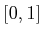and generating a dense sample sequence for any bounded C-space,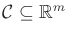. In this section the goal is to generate uniform random samples. This means that the probability density function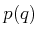overis uniform. Wherever relevant, it also will mean that the probability density is also consistent with the Haar measure. We will not allow any artificial bias to be introduced by selecting a poor parameterization. For example, picking uniform random Euler angles does not lead to uniform random samples over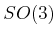. However, picking uniform random unit quaternions works perfectly because quaternions use the same parameterization as the Haar measure; both choose points on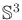.

Random sampling is the easiest of all sampling methods to apply to C-spaces. One of the main reasons is that C-spaces are formed from Cartesian products, and independent random samples extend easily across these products. If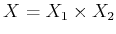, and uniform random samples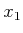and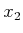are taken from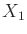and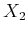, respectively, then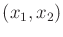is a uniform random sample for. This is very convenient in implementations. For example, suppose the motion planning problem involves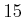robots that each translate for any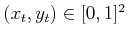; this yields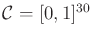. In this case,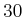points can be chosen uniformly at random fromand combined into a-dimensional vector. Samples generated this way are uniformly randomly distributed over. Combining samples over Cartesian products is much more difficult for nonrandom (deterministic) methods, which are presented in Sections 5.2.3 and 5.2.4.

Subsections
Steven M LaValle 2020-08-14